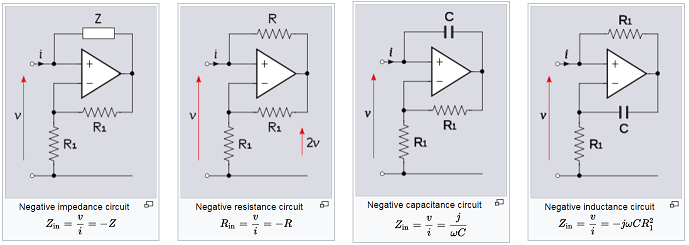# Design of Negative Impedance ConverterThe negative impedance converter (NIC) is a one-port Op-Amp circuit acting as a negative load which injects energy into circuits in contrast to an ordinary load that consumes energy from them. This is achieved by adding or subtracting excessive varying voltage in series to the voltage drop across an equivalent positive impedance. This reverses the voltage polarity or the current direction of the port and introduces a phase shift of 180° (inversion) between the voltage and the current for any signal generator. The two versions obtained are accordingly a negative impedance converter with voltage inversion (VNIC) and a negative impedance converter with current inversion (INIC). The basic circuit of an INIC is shown below. The negative of any impedance can be produced by a negative impedance converter, including negative capacitance and negative inductance.

Several application examples of negative impedance converter are shown in Fig.2. The possible applications of this converter: To eliminate the losses of a reactive circuit element, to compensate parasitic capacitance, etc.
In this project, you will design the negative capacitance converter based on the folded cascode Op-Amp.